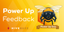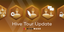# The Mathematics of geometric series (BTC like issuance)

in Proof of Brain2 years ago (edited)

The Mathematics of Bitcoin issuance and those that are Bitcoin like is what is called a Geometric series. The general form is.

∑ kaⁿ = k + ka + ka ² + ...
n=0

Here a < 1. If a = 1 or is greater, the sum diverges ( goes to infinity ). In layman's terms if the subsidy is constant or grows by some percentage instead of getting smaller then the total is ∞ (no duh). So a has to be less than one.

The letter a in the case of a 20% reduction per year results in the coin base being multiplied by 0.8 each year. This makes a=0.80. Let's suppose we start with a coinbase of 1,314,000 per year:

∑ 1,314,000 * (⁴/₅)ⁿ
n=0

Now there is a way to calculate the total sum of the series without a spreadsheet and without too much time spent with a calculator. The rule is:

∑ kaⁿ = k + ka + ka ² + ... = k / ( 1 - a) where (0 ≤ a < 1)
n=0

For this case we have:

k / ( 1 - ⁴/₅) = k / (¹/₅) = 5*k

The total supply will be five times the value of the initial subsidy period.

For Bitcoin:

k / ( 1 - ¹/₂) = k / (¹/₅) = 2*k

Half the bitcoins that will ever exist were mined during the 50 bitcoins per block period.

I used the following formula to obtain 4,200,000.
=1314000*2100/657 . I could have used the formula above total = 5k
So the initial should be one fifth of 21,000,000, which is 4,200,000.

To get from the previous subsidy to the next subsidy I put for cell C2:
=FLOOR(0.8*C1;1e-8). Then I fill down. At the end I get 20,999,999.999,997. My spreadsheet software truncs the single satoshis to zero so in reality it should be even closer to 21,000,000.

Thanks for toptal.com for compiling all of the mathcodes together here

Posted via proofofbrain.io

Sort:

Congratulations @leprechaun! You have completed the following achievement on the Hive blockchain and have been rewarded with new badge(s) :You received more than 4250 upvotes.Your next target is to reach 4500 upvotes.

If you no longer want to receive notifications, reply to this comment with the word `STOP`Feedback from the March 1st Hive Power Up DayHive Tour Update - Financial stages